cancel
Showing results for
Did you mean:Anonymous
Not applicable

## Average - Select the Period in Minutes - Like a Moving Average

Hello all!

I have a set of data like this, with a record every 5 minutes:When analyzing a period of days, for example, there is too much data and noise. I would like to "dampen" the values, so I thought about applying something like a moving average, but not quite. I would like to have the average for each "X" minutes. For example, If you set 15 minutes, the first one would average the 3 values of 00:00, 00:05 and 00:10 and so on. I would like to do this dinamically so you can set the interval on the report.

Any thoughts?

Thanks in advance!Regards.

1 ACCEPTED SOLUTIONCommunity Support

Hi @Anonymous,

>>However, when I input my data I get this error:

The error will appear when you try to divide or mod the zero, you should add a condition to filter the zero range.

>>To be honest, I can't figure out how this doesn't work because I didn't get the logic of the "AvgPerRange

Below are some detail comment of my solution.

TimeRange table is used to set the column filed of matrix. if you try to merge this part to original table, it will group data and affect the original data, so I create a new table without any relationship to calculate the column range.

Select measure is used to get the select item from current table, if you limit them to measure level, it will get the current column value from matrix.

Calculated column Time Range is used to transfer the time to number type to calculate easily.

The logic of the "AvgPerRange" measure:

MAX([TimeRange])

Get current timeRange of the current date.

e.g: 1/31/2017 00:00:00 =0, 1/31/2017 00:15:00 = 15

var minRange= INT(MAX([TimeRange])/[select])

minRange variable is used to calculate the the dynamic date range based on current column value. (Lower limit of interval)

AVERAGEX(FILTER(ALL('Sheet1'),[TimeRange]>=minRange*[select]&&[TimeRange]<(minRange+1)*[select]),[Value])

Calculate the dynamic average based on current column value.

e.g: current column value 15,  datetime 1/31/2017 01:15:00 (timerange = 75)

dynamic formula:

AVERAGEX(FILTER(ALL('Sheet1'),[TimeRange]>=5*15&&[TimeRange]<6*15),[Value])

Get the average value from all of current table which the datetime is between 1/31/2017 01:15:00 to 1/31/2017 01:30:00

var state=MOD(HOUR(MAX([Time]))*60+MINUTE(MAX(Sheet1[Time])),[select])=0

The variable which used to control the if the average value is displaied.

In summary, the full logic is : get the dynamic average based on current time range and show the value in the correct cell.

Regards,

Xiaoxin Sheng

Community Support Team _ Xiaoxin
If this post helps, please consider accept as solution to help other members find it more quickly.
15 REPLIES 15Community Support

Hi @Anonymous,

Based on my understanding, you want to get the dynamic average about the selected time, right?

If this is a case, you can refer to below formula which used to get the specify range from slicer and calculate the average based on it.

Measures:

```SelectedTime = if(HASONEVALUE(Table[Time]),VALUES(Table[Time]),BLANK())

AverageOfSelectRangePerAttr(AllDate)=
var currentAttr=LASTNONBLANK(Table[Attribute],[Attribute])
return
AVERAGEX(FILTER(ALL(Table),[Time]<[SelectedTime]&&[Attribute]=currentAttr),[Value])

AverageOfSelectRangePerAttr(CurrentDate)=
var currentAttr=LASTNONBLANK(Table[Attribute],[Attribute])
return
AVERAGEX(FILTER(ALL(Table),[Time]<[SelectedTime]&&[Attribute]=currentAttr&&[Date]=MAX(Date]),[Value])

AverageOfSelectRange(AllDate)=
AVERAGEX(FILTER(ALL(Table),[Time]<[SelectedTime]),[Value])

AverageOfSelectRange(CurrentDate)=
AVERAGEX(FILTER(ALL(Table),[Time]<[SelectedTime]&&[Date]=MAX(Date]),[Value]) ```

Regards,

Xiaoxin Sheng

Community Support Team _ Xiaoxin
If this post helps, please consider accept as solution to help other members find it more quickly.Anonymous
Not applicable

@v-shex-msft

I unpivotted the data, like this below. Is this a good step?

Thanks.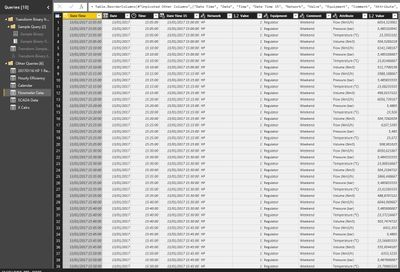Community Support

Hi @Anonymous,

Sorry for slow response, I have an idea to achieve your requirement, perhaps you can take a look at blow steps:

1. Add a custom column "Minute" to convert the time column to number.

2. Use "Minute" column which created above as the source of slicer.

3. Write the measure to get the selected Item.

`Selected Value = IF(HASONEVALUE('Table'[Minute]),VALUES('Table'[Minute]),BLANK())`

4. Calculate based on slicer and "Minute".

Measure:

```AvgPerRange=
var minRange= INT(MAX([Minute])/[Selected Value])
return
AVERAGEX(FILTER(ALL('Table'),[Minute]>=minRange*[Selected Value]&&[Minute]<(minRange+1)*[Selected Value]),[Value])```

Regards,

Xiaoxin Sheng

Community Support Team _ Xiaoxin
If this post helps, please consider accept as solution to help other members find it more quickly.Anonymous
Not applicable

@v-shex-msft

Sheng,

But I couldn't get to the value I was looking for. I only got one value instead of multiple.

Anyway I think I didn't understand your formula 100%. I was not sure what to write on [Value]. The Sum of the column I want?

To make it clearer I made an excel sheet with a piece of data and a table with the desired output. It is below. I can send the file if you want to check it. The *.pbix as well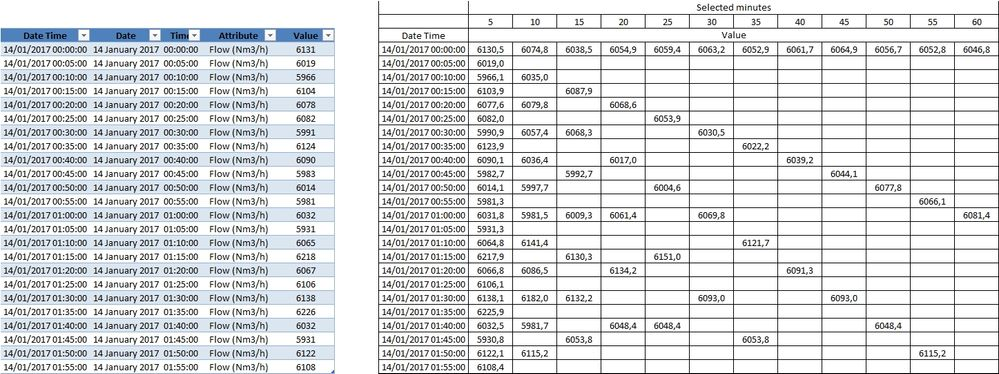Desired output

If this is taking you too long, please just don't care. I don't want to push to hard on getting helpOnce again, I am trying to get this measure to avoid the ton of values I have now.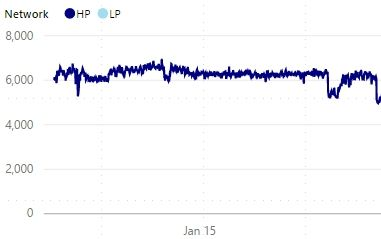Too many values

Thanks again!Community Support

Hi @Anonymous,

You can refer to below sample file if it works on your side:

Test result:Regards,

Xiaoxin Sheng

Community Support Team _ Xiaoxin
If this post helps, please consider accept as solution to help other members find it more quickly.Anonymous
Not applicable

Hello @v-shex-msft!

For your data, the solution works perfectly. However, when I input my data I get this error: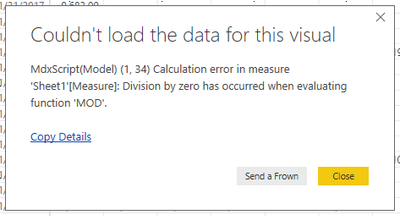Error

Then, even with your original data, when I isolate the "MOD(HOUR(MAX([Time]))*60+MINUTE(MAX(Sheet1[Time])),[select])" on a new Measure, I get the same error.

To be honest, I can't figure out how this doesn't work because I didn't get the logic of the "AvgPerRange =
var minRange= INT(MAX([TimeRange])/[select])
var state=MOD(HOUR(MAX([Time]))*60+MINUTE(MAX(Sheet1[Time])),[select])=0
return
if(state, AVERAGEX(FILTER(ALL('Sheet1'),[TimeRange]>=minRange*[select]&&[TimeRange]<(minRange+1)*[select]),[Value]),BLANK())"

Could you briefly explain me, please?

From there, maybe I can detect the error and why it doesn't work for my data.

Thanks for your help and regards.Community Support

Hi @Anonymous,

>>However, when I input my data I get this error:

The error will appear when you try to divide or mod the zero, you should add a condition to filter the zero range.

>>To be honest, I can't figure out how this doesn't work because I didn't get the logic of the "AvgPerRange

Below are some detail comment of my solution.

TimeRange table is used to set the column filed of matrix. if you try to merge this part to original table, it will group data and affect the original data, so I create a new table without any relationship to calculate the column range.

Select measure is used to get the select item from current table, if you limit them to measure level, it will get the current column value from matrix.

Calculated column Time Range is used to transfer the time to number type to calculate easily.

The logic of the "AvgPerRange" measure:

MAX([TimeRange])

Get current timeRange of the current date.

e.g: 1/31/2017 00:00:00 =0, 1/31/2017 00:15:00 = 15

var minRange= INT(MAX([TimeRange])/[select])

minRange variable is used to calculate the the dynamic date range based on current column value. (Lower limit of interval)

AVERAGEX(FILTER(ALL('Sheet1'),[TimeRange]>=minRange*[select]&&[TimeRange]<(minRange+1)*[select]),[Value])

Calculate the dynamic average based on current column value.

e.g: current column value 15,  datetime 1/31/2017 01:15:00 (timerange = 75)

dynamic formula:

AVERAGEX(FILTER(ALL('Sheet1'),[TimeRange]>=5*15&&[TimeRange]<6*15),[Value])

Get the average value from all of current table which the datetime is between 1/31/2017 01:15:00 to 1/31/2017 01:30:00

var state=MOD(HOUR(MAX([Time]))*60+MINUTE(MAX(Sheet1[Time])),[select])=0

The variable which used to control the if the average value is displaied.

In summary, the full logic is : get the dynamic average based on current time range and show the value in the correct cell.

Regards,

Xiaoxin Sheng

Community Support Team _ Xiaoxin
If this post helps, please consider accept as solution to help other members find it more quickly.Anonymous
Not applicable

@v-shex-msft

Just one more thing please, probably quite easy to solveI am having trouble applying this because I have a Calendar Table, like below. The value I am looking to apply the average is 'Flowmeter Data'[Value].Relations

So, the Tables and Columns look this this: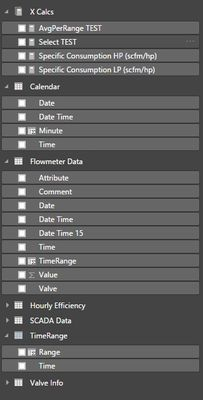Tables and Columns

Time = VALUES('Calendar'[Time])

Range = if(MINUTE([Time])=0,,60,,MINUTE([Time]))

Select TEST = if(HASONEVALUE('TimeRange'[Range]),VALUES('TimeRange'[Range]),BLANK())

TimeRange = HOUR([Time])*60+MINUTE([Time])

AvgPerRange TEST =
var minRange= INT(MAX('Flowmeter Data'[TimeRange])/[Select TEST])
var state=MOD(HOUR(MAX('Flowmeter Data'[Time]))*60+MINUTE(MAX('Flowmeter Data'[Time])),[Select TEST])=0
return
if(state, AVERAGEX(FILTER(ALL('Flowmeter Data'),[TimeRange]>=minRange*[Select TEST]&&[TimeRange]<(minRange+1)*[Select TEST]),'Flowmeter Data'[Value]),BLANK())

I can't show the data as it just calculates something and it never really appears on the table. I think this is because of the relations and the inputs on the measure AvgPerRange TEST

What do you think?

Regards.Community Support

Hi @Anonymous,

>>I can't show the data as it just calculates something and it never really appears on the table. I think this is because of the relations and the inputs on the measure AvgPerRange TEST

If you can please provide some sample file and share the link, I will check it.

Regards,

Xiaoxin Sheng

Community Support Team _ Xiaoxin
If this post helps, please consider accept as solution to help other members find it more quickly.Anonymous
Not applicable

Hello @v-shex-msft

Sure, it is attached.

Thank you AGAINCommunity Support

Hi @Anonymous,

Based on test, I find the issue is caused by total row and total column, you should use other columns to turn off these feature first, then the visual will works smoothly.

Screenshot: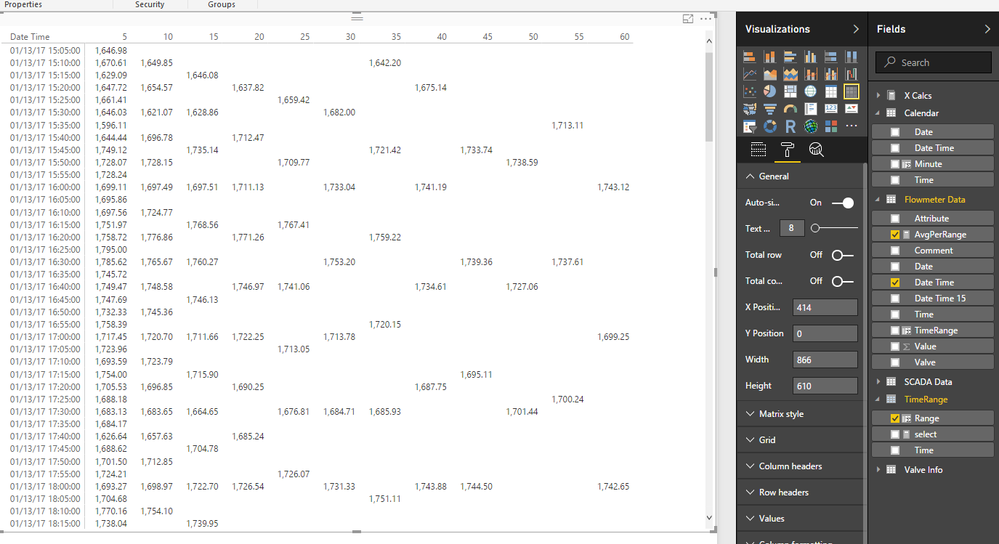BTW, I modify the measure to allow filter works on it.

```AvgPerRange =
var minRange= INT(MAX([TimeRange])/[select])
var state=MOD(HOUR(MAX([Time]))*60+MINUTE(MAX('Flowmeter Data'[Time])),[select])=0
return
if(state, AVERAGEX(FILTER(ALLSELECTED('Flowmeter Data'),[Date]=MAX([Date])&&[TimeRange]>=minRange*[select]&&[TimeRange]<(minRange+1)*[select]),[Value]),BLANK())```

Regards,

Xiaoxin Sheng

Community Support Team _ Xiaoxin
If this post helps, please consider accept as solution to help other members find it more quickly.Anonymous
Not applicable

Perfect!

Thank you so much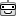The result is below.

Regards.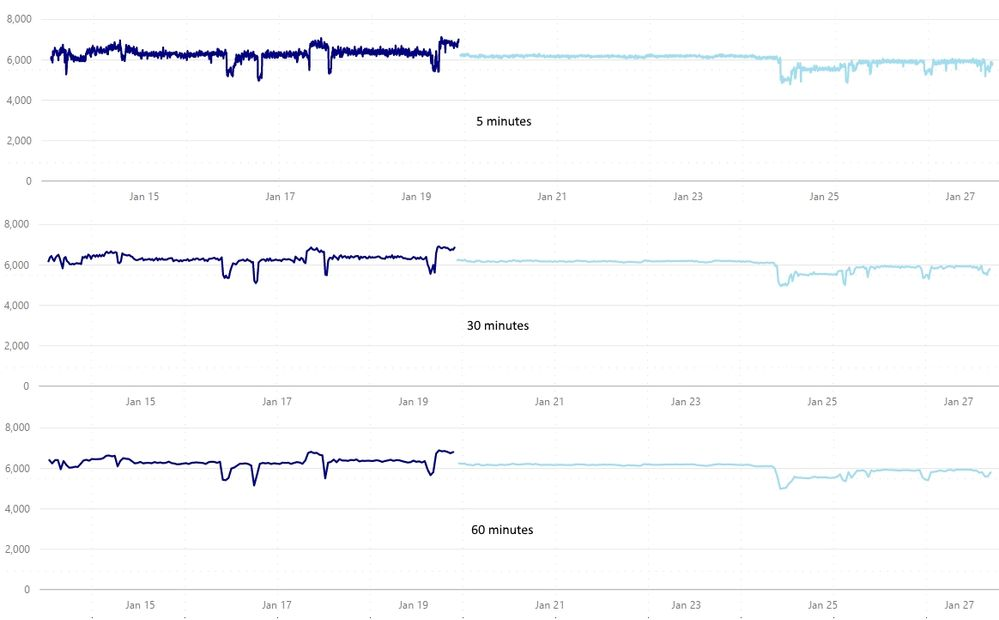SampleAnonymous
Not applicable

@v-shex-msft

Thanks a lot! I got it.

I also understood the cause for the MOD error... It was due to the Range = if(MINUTE([Time])=0,60,MINUTE([Time]))

Stupid error. I don't know why but on DAX, to input a value I have to put the decimal separator (",") even if it is an integer. This breaks my balls. Like this: Range = if(MINUTE([Time])=0,,60,,MINUTE([Time]))

Thanks, I will use this techniques for other Dashboards, most certainly!Anonymous
Not applicable

Any thoughts on this, anyone?Anonymous
Not applicable

Sheng,

I tried your measures but that didn't work as expected, and I didn't understand how it works as well. Let me give you more info, I think it will be easier to understand.

First let me show you the relationships.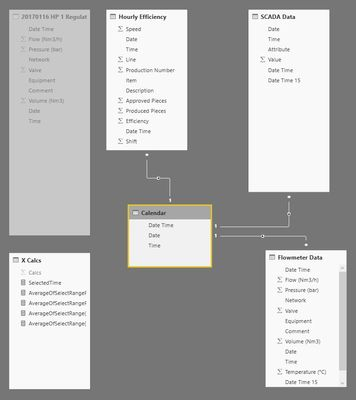I have, among others, 2 tables that I would like to relate.

Those being 'Flowmeter Data' and 'SCADA Data'.

In 'SCADA Data', the values are unpivotted by [Attibute].

In 'Flowmeter Data', each line is a value with multiple columns.

BTW, should I unpivotted this for the sake of data organization?

So I would like to do this operation that I mentioned for the 2 tables. For example, if I select 5 minutes the data would not be changed but if I selected 30 minutes, I would get the average from 00:00 to 00:25, then 00:30 to 00:55, and so on.

Maybe there's something you can add now?

ThanksAnnouncements#### Exclusive opportunity for Women!

Join us for a free, hands-on Microsoft workshop led by women trainers for women where you will learn how to build a Dashboard in a Day!#### Power Platform Conference-Power BI and Fabric Sessions

Join us Oct 1 - 6 in Las Vegas for the Microsoft Power Platform Conference.Top Solution Authors
Top Kudoed Authors
Users online (1,949)